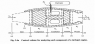# Aeroengines/Fluid Mechanics - Steady Flow

JRS192

## Homework Statement

See Fig.2.4a, let the gases flowing through the nozzle control volume be perfect with Cp = 1.004kJ/kg.K

Determine V9 if T5 = 1000K, T9 = 670K and V5 = 120ms^-1.

## Homework Equations

I've detailed the equations in the attached pdf for clarity.

## The Attempt at a Solution

See attached pdf. The question paper has the answer as 822.8m/s, but I'm consistently getting 120m/s. Where am I going wrong?

Thank you

#### Attachments

•Fig2.4a.png
47.3 KB · Views: 437
• doc00586420150126151206.pdf
329.4 KB · Views: 159

Staff Emeritus
Homework Helper
You've confused the universal gravitational constant G for the acceleration due to gravity on earth, gc.
It's not clear why you don't understand the distinction between these two constants.

JRS192
Hi SteamKing,

Thanks for that. I did some research and everything directed me to that figure, even to the point of using gc...

Staff Emeritus
Homework Helper
Hi SteamKing,

Thanks for that. I did some research and everything directed me to that figure, even to the point of using gc...

I doubt that. You have misinterpreted something in what you read.

The universal gravitational constant G (which is invariably capitalized) is typically used to find the force of gravitational attraction between two masses, as described by the formula

F = Gm1m2/r2

G is used more often in physics type calculations. G also has some rather unusual units: G = 6.67*10-11 m3/kg-s2

The acceleration due to gravity on earth, gc, which is invariably lower case, is typically commonly found in engineering type calculations, more so than G.

JRS192